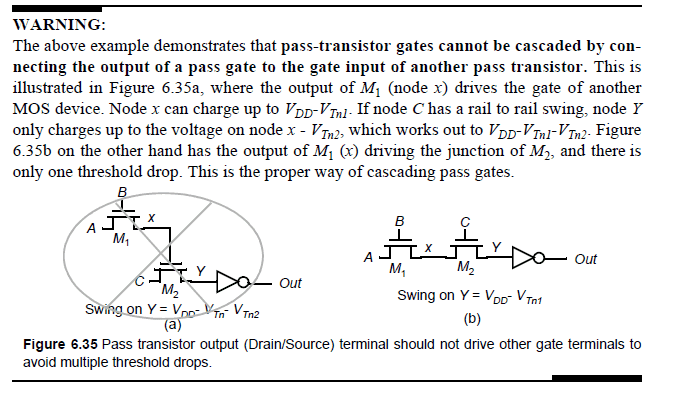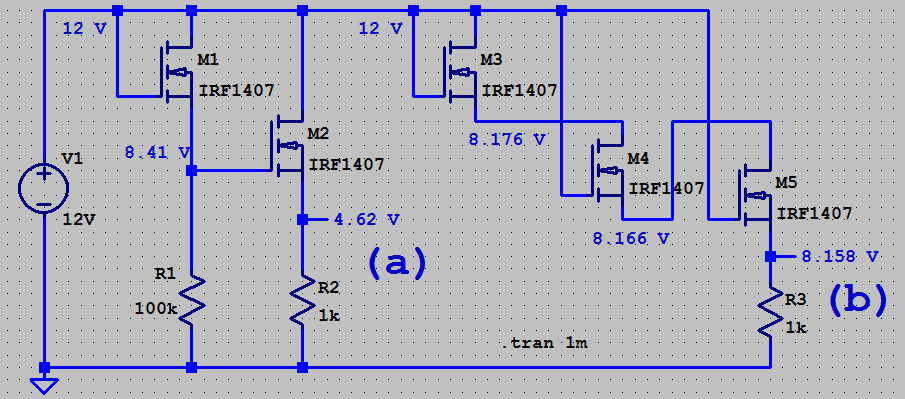# Voltage Swing for NMOS

Summary:
Voltage swing for nmos is Vdd - Vth? How?Ref: Digital Integrated Circuits by Jan M. Rabaey

I have trouble understanding two things:
1) Why will x charge to Vdd - Vtn1 and why not Vdd?
2) If x in left charges to Vdd - Vtn1, then in right also it would charge to Vdd - Vtn1... so Vy would charge to Vdd - Vtn1 - Vtn2... so why have they written the swing as vdd - Vtn1 and why not Vdd - Vtn1 - Vtn2?

Baluncore
1. When A and B are connected to Vdd, M1 is a source follower, if the source X then rises above Vdd–Vth, M1 will turn off and so X will stop rising

2. Both M1 and M2 are source followers with their source voltages set between common and Vdd–Vth. The voltage dropped across M2 can be zero so Vth is not cascaded.

•jaus tail
Thanks. I understood the 1 part. But not the second part. How will drop across M2 be zero. Drop across M2 is overdrive voltage, right? I.e Vds.

Vx can be Vdd - Vth. If Vy also becomes Vdd-Vth, then there will be no current flow as Vds = 0. No potential difference so no current flow.

Baluncore
Think of an NMOS FET as a variable resistor, with Ron set by Vgs. The voltage drop across M2 = Vsd, will depend on output load current multiplied by Ron of M2. For low currents it will be only a few mV, so close to zero.

Ok, but if Vds is less than Vov, then the mosfet would go in linear mode, right? That's not a favorable mode (I don't know why though. Most switch mode should be cut off or saturation)

Baluncore